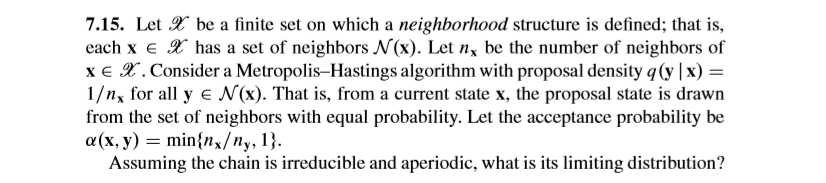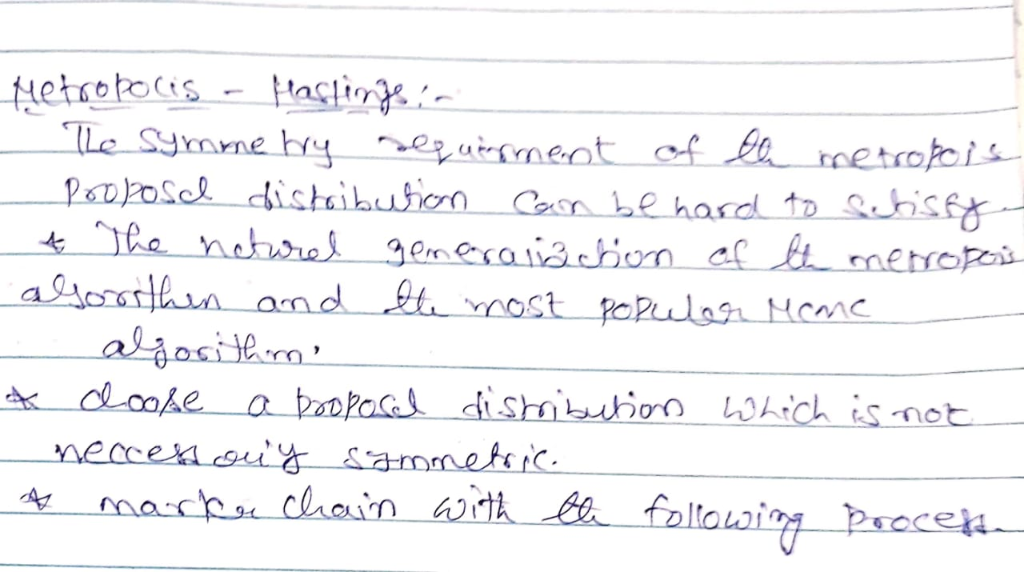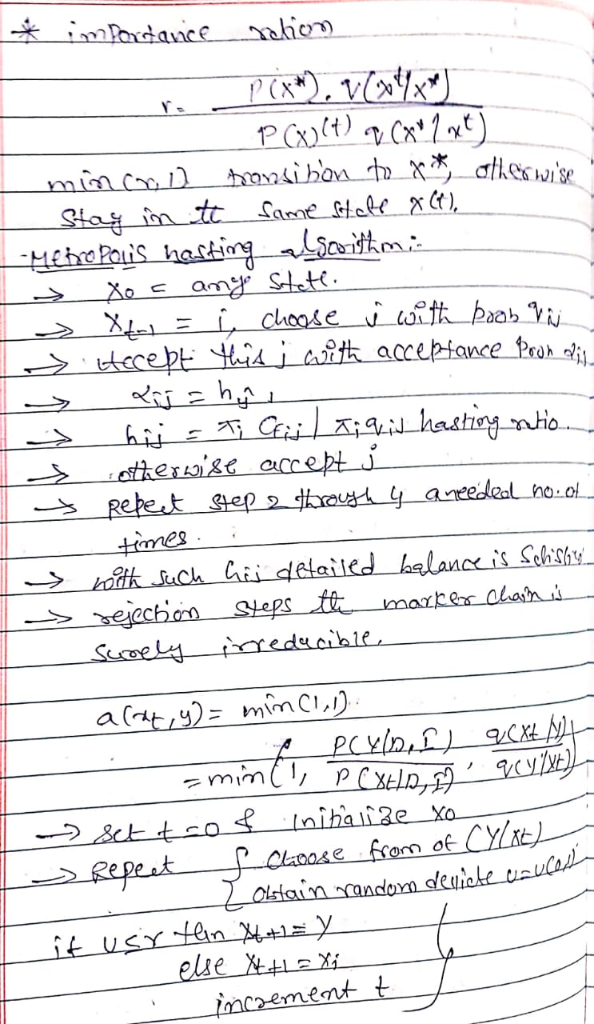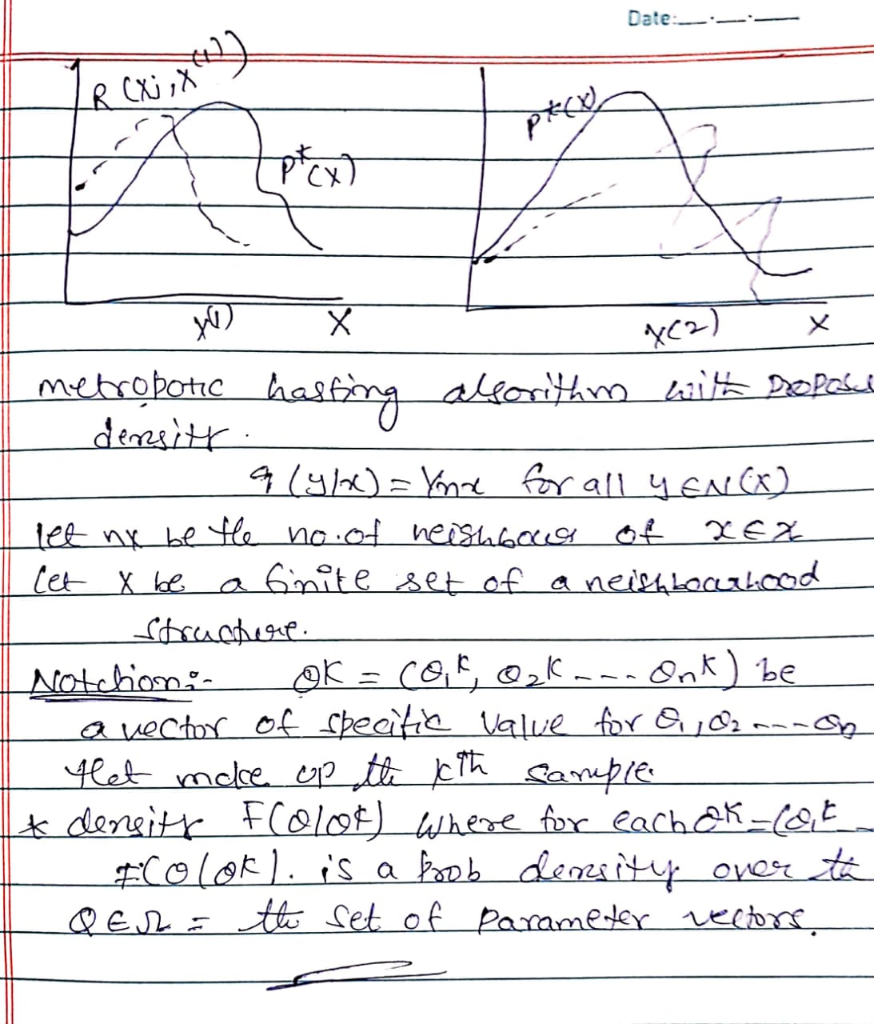# Please do not copy the answers from the same question. I dont understand that one! And full steps please. 7.15. Let be...Please do not copy the answers from the same question. I dont understand that one! And full steps please.

7.15. Let be a finite set on which a neighborhood structure is defined; that is, each x E has a set of neighbors N(x). Let nx be the number of neighbors of x E . Consider a Metropolis-Hastings algorithm with proposal density q(y |x) - 1/n for all y E N(x). That is, from a current state x, the proposal state is drawn from the set of neighbors with equal probability. Let the acceptance probability be Assuming the chain is irreducible and aperiodic, what is its limiting distribution?More Homework Help Questions Additional questions in this topic.